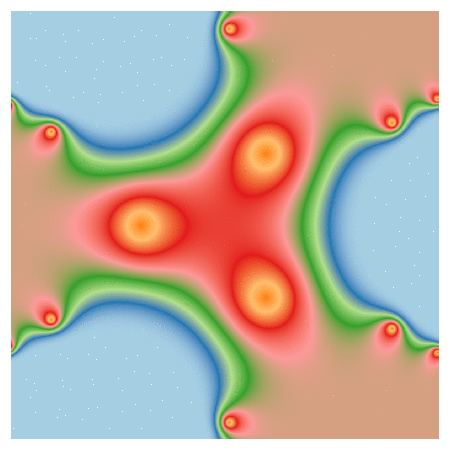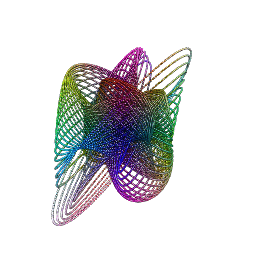# Playing With Julia (Set)

Viento, me pongo en movimiento y hago crecer las olas del mar que tienes dentro (Tercer Movimiento: Lo de Dentro, Extremoduro)

I really enjoy drawing complex numbers: it is a huge source of entertainment for me. In this experiment I play with the Julia Set, another beautiful fractal like this one. This is what I have done:

• Choosing the function `f(z)=exp(z3)-0.621`
• Generating a grid of complex numbers with both real and imaginary parts in [-2, 2]
• Iterating `f(z)` over the grid a number of times so `zn+1 = f(zn)`
• Drawing the resulting grid as I did here
• Gathering all plots into a GIF with ImageMagick as I did in my previous post: each frame corresponds to a different number of iterations

This is the result:I love how easy is doing difficult things in R. You can play with the code changing `f(z)` as well as color palettes. Be ready to get surprised:

```library(ggplot2)
library(dplyr)
library(RColorBrewer)
dir.create("output")
setwd("output")
f = function(z,c) exp(z^3)+c
# Grid of complex
z0 <- outer(seq(-2, 2, length.out = 1200),1i*seq(-2, 2, length.out = 1200),'+') %>% c()
opt <-  theme(legend.position="none",
panel.background = element_rect(fill="white"),
plot.margin=grid::unit(c(1,1,0,0), "mm"),
panel.grid=element_blank(),
axis.ticks=element_blank(),
axis.title=element_blank(),
axis.text=element_blank())
for (i in 1:35)
{
z=z0
# i iterations of f(z)
for (k in 1:i) z <- f(z, c=-0.621) df=data.frame(x=Re(z0), y=Im(z0), z=as.vector(exp(-Mod(z)))) %>% na.omit()
p=ggplot(df, aes(x=x, y=y, color=z)) +
geom_tile() +
scale_x_continuous(expand=c(0,0))+
scale_y_continuous(expand=c(0,0))+
ggsave(plot=p, file=paste0("plot", stringr::str_pad(i, 4, pad = "0"),".png"), width = 1.2, height = 1.2)
}
# Place the exact path where ImageMagick is installed
system('"C:\\Program Files\\ImageMagick-6.9.3-Q16\\convert.exe" -delay 20 -loop 0 *.png julia.gif')
# cleaning up
file.remove(list.files(pattern=".png"))
```

# Zooming

You don’t have to be beautiful to turn me on (Kiss, Prince)

I discovered recently how easy is to create GIFs with R using ImageMagick and I feel like a kid with a new toy. To begin this new era of my life as R programmer I have done this:First of all, read this article: it explains very well how to start doing GIFs from scratch. The one I have done is inspired in this previous post where I take a set of complex numbers to transform and color it using HSV technique. In this case I use this next transformation:$f(z)= -Im(z)+(Re(z)+0.5*Im(z))*1i$

Modifying the range of `Real` and `Imaginary` parts of complex numbers I obtain the zooming  effect. The code is very simple. Play with it changing the transformation or the animation options. Send me your creations, I would love to see them:

```library(dplyr)
library(ggplot2)
dir.create("output")
setwd("output")
id=1 # label tO name plots
for (i in seq(from=320, to=20, length.out = 38)){
z=outer(seq(from = -i, to = i, length.out = 300),1i*seq(from = -i, to = i, length.out = 500),'+') %>% c()
z0=z
for (k in 1:100) z <- -Im(z)+(Re(z)+0.5*Im(z))*1i
df=data.frame(x=Re(z0),
y=Im(z0),
h=(Arg(z)<0)*1+Arg(z)/(2*pi), s=(1+sin(2*pi*log(1+Mod(z))))/2, v=(1+cos(2*pi*log(1+Mod(z))))/2) %>% mutate(col=hsv(h,s,v))
ggplot(df, aes(x, y)) +
geom_tile(fill=df\$col)+
scale_x_continuous(expand=c(0,0))+
scale_y_continuous(expand=c(0,0))+
labs(x=NULL, y=NULL)+
theme(legend.position="none",
panel.background = element_rect(fill="white"),
plot.margin=grid::unit(c(1,1,0,0), "mm"),
panel.grid=element_blank(),
axis.ticks=element_blank(),
axis.title=element_blank(),
axis.text=element_blank())
id=id+1
}
system('"C:\\Program Files\\ImageMagick-6.9.3-Q16\\convert.exe" -delay 10 -loop 0 -duplicate 1,-2-1 *.png zooming.gif')
# cleaning up
file.remove(list.files(pattern=".png"))
```

# 3D-Harmonographs In Motion

I would be delighted to co write a post (Andrew Wyer)

One of the best things about writing a blog is that occasionally you get to know very interesting people. Last October 13th I published this post about the harmonograph, a machine driven by pendulums which creates amazing curves. Two days later someone called Andrew Wyer made this comment about the post:

Hi, I was fascinated by the harmonographs – I remember seeing similar things done on paper on kids tv in the seventies. I took your code and extended it into 3d so I could experiment with the rgl package. I created some beautiful figures (which I would attach if this would let me). In lieu of that here is the code:

I ran his code and I was instantly fascinated: resulting curves were really beautiful. I suggested that we co-write a post and he was delighted with the idea. He proposed to me the following improvement of his own code:

I will try to create an animated gif of one figure

Such a good idea! And no sooner said than done: Andrew rewrote his own code to create stunning animated images of 3D-Harmonograph curves like these:• Andrew creates 3D curves by adding a third oscillation z generated in the same way as x and y and adds a little colour by setting the colour of each point to a colour in the RGB scale related to its point in 3d space
• Function `spheres3d `to produce an interactive plot that you can drag around to view from different angles; function `spin3d `will rotate the figure around the z axis and at 5 rpm in this case and function `movie3d `renders each frame in a temporary png file and then calls ImageMagick to stitch them into an animated gif file. It is necessary to install ImageMagick separately to create the movie.
• Giving it a duration of 12 seconds at 5 rpm is one rotation which at 12 frames per second results in 144 individual png files but these (by default) are temporary and deleted when the gif is produced.

Although I don’t know Andrew personally, I know he is a good partner to work with. Thanks a lot for sharing this work of art with me and allowing me to share it in Ripples as well.

Here you have the code. I like to imagine these curves as orbits of unexplored planets in a galaxy far, far away …

```library(rgl)
library(scatterplot3d)
#Extending the harmonograph into 3d
#Antonio's functions creating the oscillations
xt = function(t) exp(-d1*t)*sin(t*f1+p1)+exp(-d2*t)*sin(t*f2+p2)
yt = function(t) exp(-d3*t)*sin(t*f3+p3)+exp(-d4*t)*sin(t*f4+p4)
#Plus one more
zt = function(t) exp(-d5*t)*sin(t*f5+p5)+exp(-d6*t)*sin(t*f6+p6)
#Sequence to plot over
t=seq(1, 100, by=.001)
#generate some random inputs
f1=jitter(sample(c(2,3),1))
f2=jitter(sample(c(2,3),1))
f3=jitter(sample(c(2,3),1))
f4=jitter(sample(c(2,3),1))
f5=jitter(sample(c(2,3),1))
f6=jitter(sample(c(2,3),1))
d1=runif(1,0,1e-02)
d2=runif(1,0,1e-02)
d3=runif(1,0,1e-02)
d4=runif(1,0,1e-02)
d5=runif(1,0,1e-02)
d6=runif(1,0,1e-02)
p1=runif(1,0,pi)
p2=runif(1,0,pi)
p3=runif(1,0,pi)
p4=runif(1,0,pi)
p5=runif(1,0,pi)
p6=runif(1,0,pi)
#and turn them into oscillations
x = xt(t)
y = yt(t)
z = zt(t)
#create values for colours normalised and related to x,y,z coordinates
cr = abs(z)/max(abs(z))
cg = abs(x)/max(abs(x))
cb = abs(y)/max(abs(y))
dat=data.frame(t, x, y, z, cr, cg ,cb)
#plot the black and white version
with(dat, scatterplot3d(x,y,z, pch=16,cex.symbols=0.25, axis=FALSE ))
with(dat, scatterplot3d(x,y,z, pch=16, color=rgb(cr,cg,cb),cex.symbols=0.25, axis=FALSE ))
#Set the stage for 3d plots
# clear scene:
clear3d("all")
# white background
bg3d(color="white")
#lights...camera...
light3d()
#action
# draw shperes in an rgl window